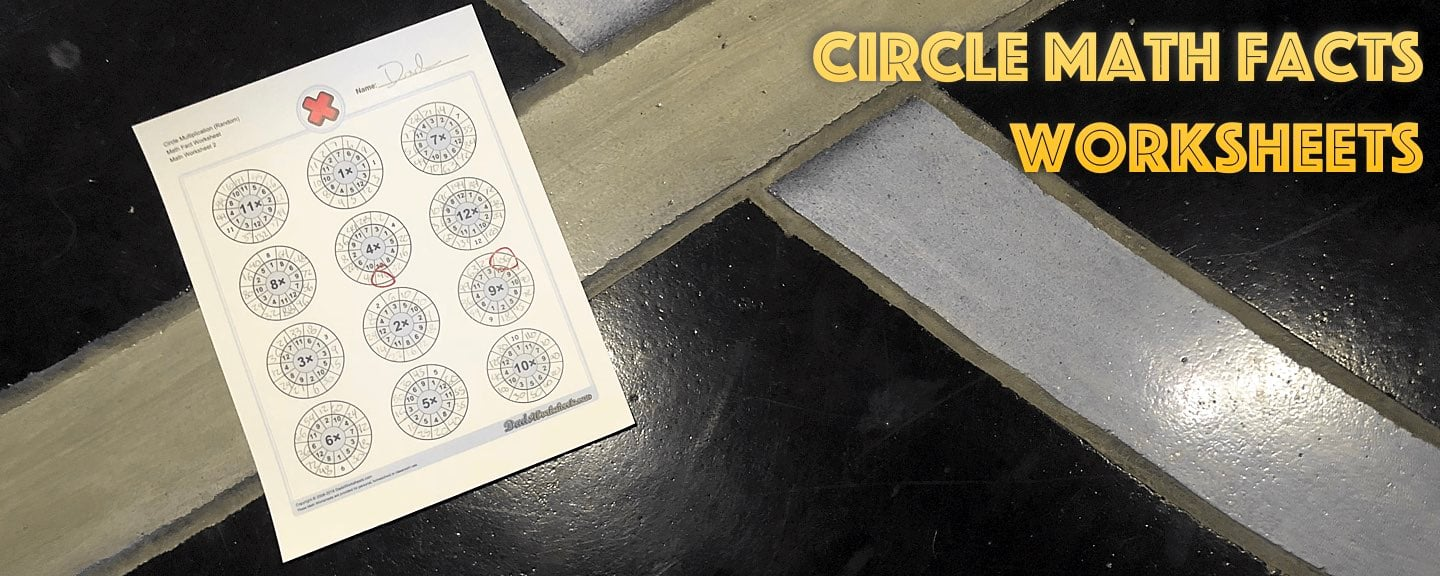One Dad. Four daughters. 9,393 worksheets... and counting!# Need Another Spin on Math Facts? Try These New Circle Worksheets!

There are never too many ways to practice math facts, and these new circle worksheets are another approach to learning how numbers are related to each other in addition, subtraction, multiplication or division groups!

Each circle collects a set of math facts related to a single number, for example the multiplication worksheets all have a common value, so each circle is essentially a set of multiplication facts. You can go around the circle and solve each fact to help emphasize strategies like skip counting or repeated addition to learn multiplication. Alternatively, some of the worksheets have the facts in a random order around the circle which requires students to think a bit more to solve the whole set.

The addition, subtraction and division worksheets all follow a similar pattern. When you’re working to establish the relationships between similar sets of math facts for these operations, you’ll find these worksheets to be another useful tool, and I hope a visually interesting one as well!

If you have an idea for more worksheets, let me know in the comments. And as always, I appreciate your sharing these (or any other worksheets) with other parents or educators who would find them useful!

I hope your autumn is off to a great start!